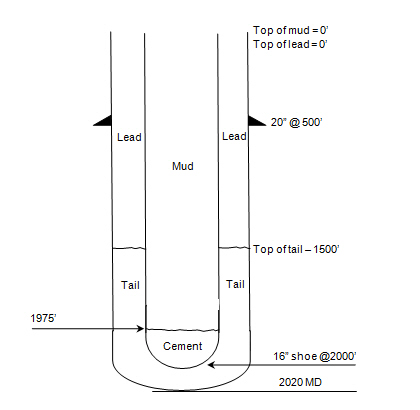## Pressure Loss and Equivalent Circulating Density Review – Reverse Circulation

We still have the same question as the previous post, Pressure Loss and Equivalent Circulating Density Review, but this case we will do reverser circulation, circulating from annulus to tubing , and see how much pressure and equivalent circulating density at bottom hole.## Pressure Loss and Equivalent Circulating Density Review

This example that I got from my junior member is very simple but it helps you a lot to understand about how to determine pressure loss during normal circulation.

Information given is listed below;Circulate at 3 bottom up through open end tubing (Down tubing and up annulus) with 12.7 ppg mud.

Pump pressure = 1000 psi

Annulus friction loss = 50 psi

Inside tubing friction loss = 925 psi

Surface line friction loss = 25 psi

## How much pressure will we see at cement head in case of float shoe fail?

I still have the simple but interesting question about hydrostatic pressure which you can apply this calculation into drilling/cementing operation. The question I got about how much pressure we will see at cement head in case of float shoe fail.## Pressure Required to Break Circulation in Annulus

From the previous post, we learn about how to determine break circulating pressure inside drill string. This post we will learn about how to calculate pressure required to break circulation in annulas.

Formula to calculate pressure required overcoming the mud’s gel strength in the annulus as follow:

Pgs = y ÷ [300 x (Dh, in. – Dp, in.)] x L

where Pgs = pressure required to break gel strength, psi

L = length of drill string, ft

y = 10 mm. gel strength of drilling fluid, lb/100 sq ft

Dh = hole diameter, in.

Dp = pipe diameter, in.

Let’s take a look at the example below and understand how to determine pressure required to break circulation in the annulus by using following information

L = 11,500 ft

y = 12 lb/100 sq ft

Dh = 6.5 in.

Dp = 4.0 in.

Referring to the formula above, all parameters can simply input into the formula to get the break circulation pressure in the annulus.

Pgs = 12 ÷ [300 x (6.5 – 4.0)] x 11,500 ft

Pgs = 184.0 psi

Please find the Excel sheet for calculating the pressure required for break circulation in the annulus.